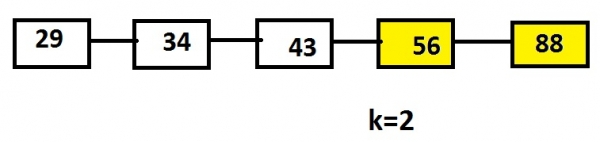# Print the last k nodes of the linked list in reverse order Recursive Approaches in C language

The task is to print the k nodes starting from the end of the linked list using recursive approach.

Recursive approach is the one in which the function call itself again and again till the call is being made and hence stores the result.

Let’s say, list contains the nodes 29, 34, 43, 56 and 88 and the value of k is 2 than the output will be the last k nodes such as 56 and 88.## Example

Linked List: 29->34->43->56->88
Input: 2
Output: 88 56

As specified the recursive approach should be used which will traverse the list from the end keeping track of the number of times the list is to be traversed and the recursive function will be called till the kth value by a pointer variable.

The below code shows the c implementation of the algorithm given.

## Algorithm

START
Step 1 -> create node variable of type structure
Declare int data
Declare pointer of type node using *next
Step 2 -> Declare function as node* get(int data)
Create newnode using malloc function
Set newnode->data = data
Set newnode->next = NULL
return newnode
step 3 -> Declare function void lastval(node* head, int& count, int k)
Return
Set count++
IF (count <= k)
Step 4 -> In Main()
Set k and count to 0
STOP

## Example

#include<stdio.h>
#include<stdlib.h>
// Structure of a node
struct node {
int data;
node* next;
};
// Function to get a new node
node* get(int data) {
struct node* newnode = (struct node*)malloc(sizeof(struct node));
newnode->data = data;
newnode->next = NULL;
return newnode;
}
//print the last k values of a node
void lastval(node* head, int& count, int k) {
return;
count++;
if (count <= k)
}
int main() {
node* head = get(11); //inserting elements into the list
int k = 2, count = 0;
printf(" last %d nodes of a list are :",k);
// print last k nodes
}
last 2 nodes of a list are :522 421